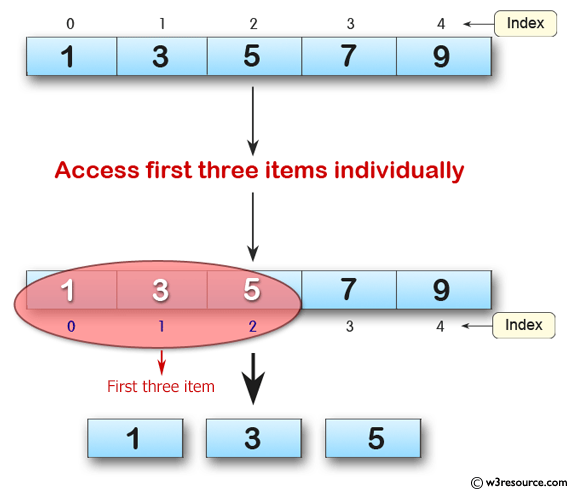﻿ Python: Create an array of 5 integers and display the array items - w3resource# Python: Create an array of 5 integers and display the array items

## Python: Array Exercise-1 with Solution

Write a Python program to create an array of 5 integers and display the array items. Access individual element through indexes.

Pictorial Presentation:Sample Solution:

Python Code :

``````from array import *
array_num = array('i', [1,3,5,7,9])
for i in array_num:
print(i)
print("Access first three items individually")
print(array_num)
print(array_num)
print(array_num)
``````

Sample Output:

```1
3
5
7
9
Access first three items individually
1
3
5
```

Python Code Editor:

Previous: Python Array Exercise Home.
Next: Write a Python program to append a new item to the end of the array.

What is the difficulty level of this exercise?

Test your Python skills with w3resource's quiz

﻿

## Python: Tips of the Day

Python: Cache results with decorators

There is a great way to cache functions with decorators in Python. Caching will help save time and precious resources when there is an expensive function at hand.

Implementation is easy, just import lru_cache from functools library and decorate your function using @lru_cache.

```from functools import lru_cache

@lru_cache(maxsize=None)
def fibo(a):
if a <= 1:
return a
else:
return fibo(a-1) + fibo(a-2)

for i in range(20):
print(fibo(i), end="|")

print("\n\n", fibo.cache_info())
```

Output:

```0|1|1|2|3|5|8|13|21|34|55|89|144|233|377|610|987|1597|2584|4181|

CacheInfo(hits=36, misses=20, maxsize=None, currsize=20)```